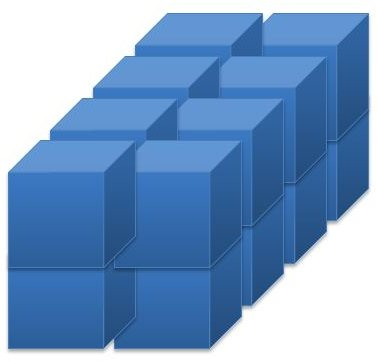Lesson Plan on Volume Measurement: Relating the Counting of Unit Cubes to Multiplication and Addition – Grade 5 Math

Concepts Covered: Measure volume of a rectangular figure by counting unit cubes, using cubic cm, cubic in, and cubic ft. Relate finding volume of a rectangular figure through counting unit cubes to multiplying the edge lengths of the rectangular figure. Also relate finding volume by multiplying the area of the base of the rectangular figure by the height.

Lesson Objective: The lesson is aligned to the Common Core State Standards for Mathematics – 5MD.5a Geometric Measurement – Relate volume to the operations of multiplication and addition and solve real world and mathematical problems involving volume.

Materials Required: unit cubes using cubic cm, cubic in, cubic ft

Lesson ProcedureThe rectangular figure on the right is packed with unit cubes with a volume of one centimeter. There are no gaps or overlaps.

1. Count the number of cubes. What is the volume of the rectangular figure?

2. What are the length, width, and height of the rectangular figure?

3. Multiply the length, width, and height. What is the product?

4. Choose the word(s) to complete the sentence:

The product of the length, width, and height of a rectangular figure _______ (is more than, is less than, equals) the volume of the rectangular figure.

Look at the rectangular figure again and answer the questions.

5. What is the area of the base of the rectangular figure?

6. What is the height of the rectangular figure?

7.Multiply the area of the base by the height. What is the product?

8. Choose the word(s) to complete the sentence:

The product of the area of the base and the height of a rectangular figure _______ (is more than, is less than, equals) the volume of the rectangular figure.

1. 16 cubic centimeters
2. 2 centimeters, 4 centimeters, 2 centimeters
3. 16 cubic centimeters
4. equals
5. 8 square centimeters
6. 2 centimeters
7. 16 cubic centimers
8. equals

Individual or Group Work

Look at Figure 1. The figure is packed with unit cubes with a volume of one cubic inch.

1. How many unit cubes are packed in this figure? What is the volume in cubic inches?

2. What are the length, width, and height?

3. Multiply the length, width, and height. What is the product?

4. What is the area of the base?

5. Multiply the area of the base by the height. What is the product?

Look at Figure 2. The figure is packed with unit cubes with a volume of one cubic centimeter.

6. How many unit cubes are packed in this figure? What is the volume in cubic centimeters?

7. What are the length, width, and height?

8. Multiply the length, width, and height. What is the product?

9. What is the area of the base?

10. Multiply the area of the base by the height. What is the product?

11. Write a sentence that compares the number of unit cubes packed into a rectangular figure to the product of the length, width, and height of the rectangular figure.

12. Write a sentence that comares the number of unit cubes packed into a recangular figure to the product of the area of the base and the height of the rectangular figure.

13. The length of a rectangular box is 4 inches, the width is 3 inches, and the height is 5 inches. How many unit cubes with a volume of one cubic inch can be packed into the rectangular box without any gaps or overlaps?

14. The length of a rectangular box is 2 inches, the width is 4 inches, and the height is 6 inches. What is the volume of the rectangular box?

15. The measurements of a rectangular box is as follows:

Length: 3 centimeters

Width: 2 centimeters

Height: 6 centimeters

What is the volume of the rectangular box? Describe in words or numbers three different ways you can use to find the volume of the rectangular box.

1. 8 cubic inches
2. 2 inches, 2 inches, 2 inches
3. 8 cubic inches
4. 4 square inches
5. 8 cubic inches
6. 18 cubic centimeters
7. 2 centimeters, 3 cenitmeters, 3 centimeters
8. 18 cubic centimeters
9. 6 square centimeters
10. 18 cubic centimeters
11. The number of unit cubes packed into a rectangular figure without gaps or overlaps equals the product of the length, width, and height of the rectangular figure.
12. The number of unit cubes packed into a rectangular figure without gaps or overlaps equals the product of the area of the base and the height of the rectangular figure.
13. 60 unit cubes
14. 48 cubic inches
15. The volume is 36 cubic centimeters.
• To find the volume, I can count the number of unit cubes I can pack into the rectangular box without gaps or overlaps.
• To find the volume, I can multiply the length, width, and height. 3 x 2 x 6 = 36 cubic centimeters.
• To find the volume, I can find the area of the base. Then multiply the area by the height. The area of the base is 3 x 2 = 6 square centimeters. Then, 6 x 6 = 36 cubic centimeters.

Students should be able to relate the number of unit cubes used to fill a rectangular figure to the product of the length, width, and height of the rectangular figure. Students should also be able to relate the volume of a rectangular figure to the product of the area of the base and the height of the rectangular figure.